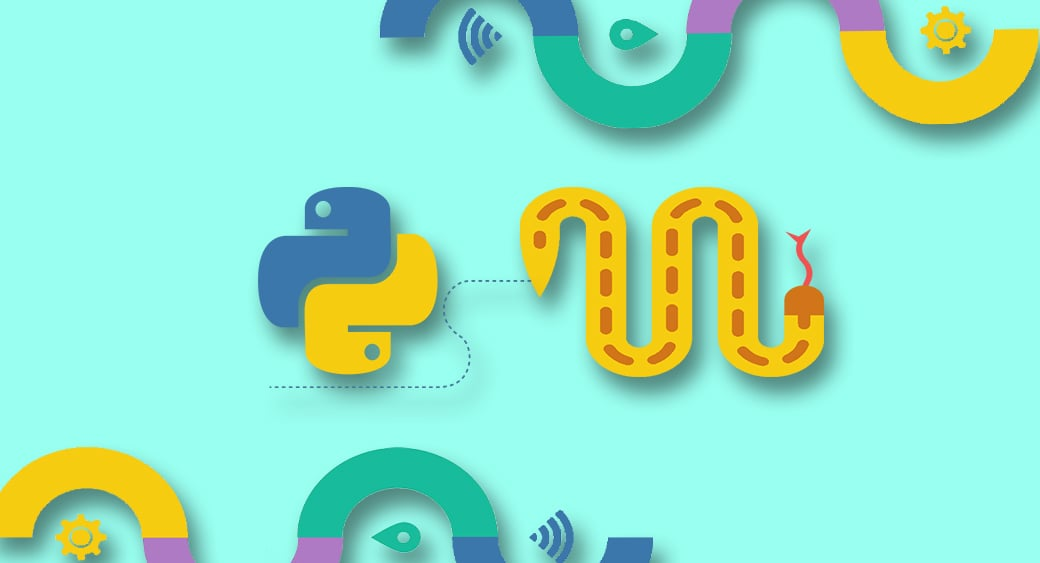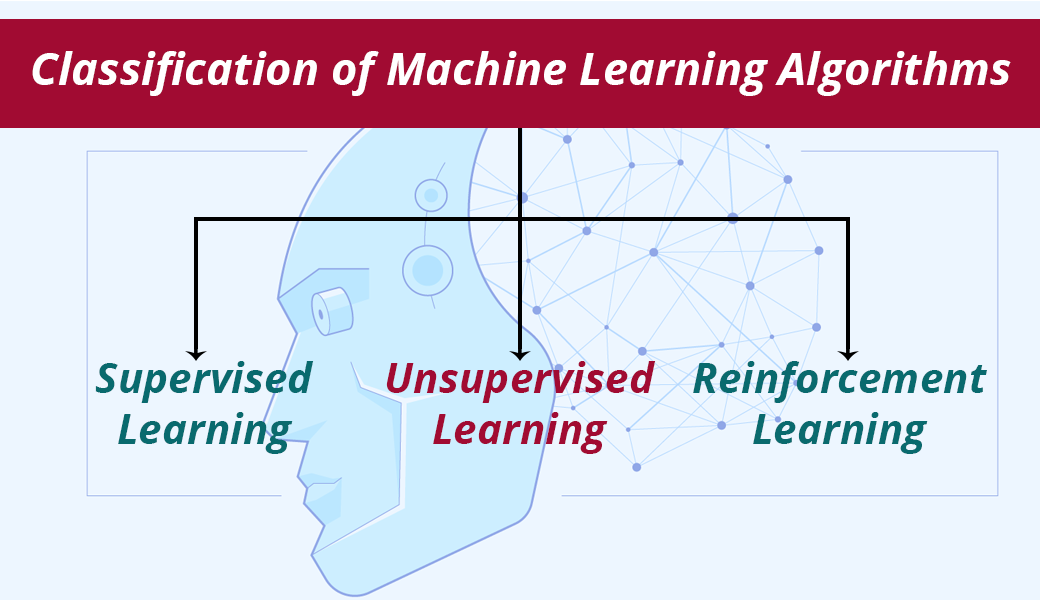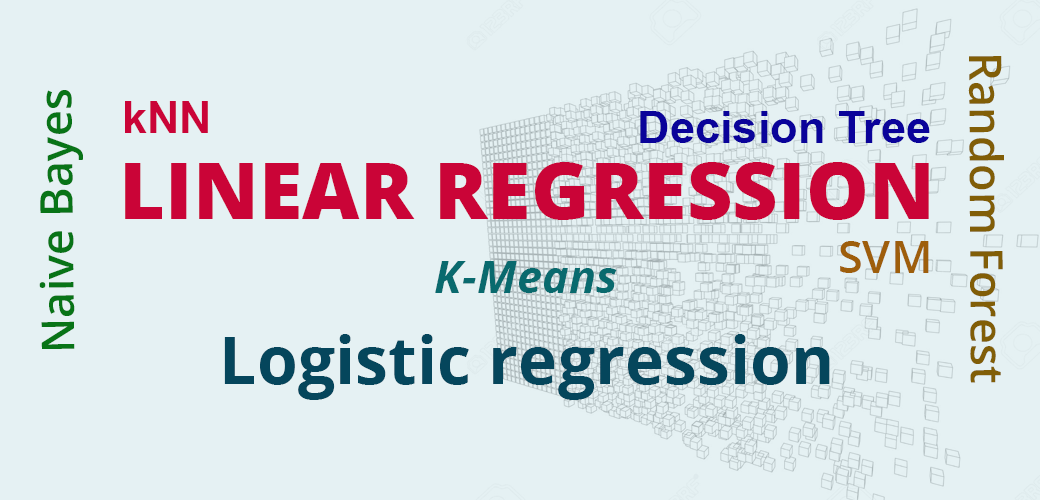# Machine Learning Algorithms in Python – Beginner`s Guide

25 May, 2018 1,332 0

Machine learning and artificial intelligence are going to be the next big thing. Global leaders in technology like Google, Facebook, Netflix have come to know about the importance of machine learning. Machine learning algorithms in Python are making machines more intelligent and smarter. Building machine learning algorithms is not an easy task. It takes days and nights of data scientists to create a functional machine learning algorithm.This article is a beginner guide to the aspiring data scientists who are willing to create a career as a Data Scientist. Python is the top choice of data scientists in building the machine learning algorithms. Here we will be discussing various machine learning algorithms in Python. This is basically a machine learning tutorial in python.

## Classification of Machine Learning Algorithms in Python

There are three types of machine learning algorithms in Python. Let us discuss them in detail.### Supervised Learning

The supervised learning consists of outcome variables that are predicted from a universal set of predictors. Supervised learning variables generate a function that maps inputs to the required outputs. The training is continued until the algorithm reaches desired degree of precision. Various examples of supervised learning are KNN, regression, logistic regression etc. Python machine learning has helped in implementing the supervised learning algorithms with much accuracy.

### Unsupervised Learning

Unlike supervised learning, it does not have target or outcomes to predict. It just classifies the population into different sections. This helps in the customer segmentation. Unsupervised learning is the most commonly used machine learning algorithm in Python. Some examples are K-means and Apriori algorithm.

### Reinforcement Learning

Another machine learning algorithm in Python that is widely used is reinforcement learning. It enables machines to take specific decisions. The machines are exposed to the environment where they can learn autonomously by hit and trial method. These have the ability to learn from experience also. Markov Decision Process is the best example. With the help of Python machine learning, reinforcement learning implementation has become easier.

Most popular machine learning algorithms: Here is a list of some of the most popular machine learning algorithms in Python:

1. Linear Regression
2. Logistic regression
3. Decision Tree
4. SVM
5. Naive Bayes
6. kNN
7. K-Means
8. Random Forest

### Linear RegressionLinear regression is a machine learning algorithm in Python that can estimate the real values. For example, cost of a house and sales of the organization. A relationship is established between independent and dependent variables. This relation is then represented in the form of the equation. For the better understanding of Linear regression, take an example of a child who is asked to arrange his classmates in the ascending order according to their weight without knowing their weight. The child would visually analyze the height, the physique of a classmate and arrange them in the ascending order. Linear regression works in the same way.

### Logistic Regression

Logistic regression is a machine learning algorithm in Python that works on discrete values like 0 and 1. It predicts the probability of an event occurrence by adjusting the discrete values. It is also known as logit regression. As it predicts the probability, its value lies between 0 and 1. For example, you are given a puzzle to solve. Either you can solve it or you are not able to solve it. Now, you are given a large number of puzzles to solve to check which subject you are good at. The output would be something like – if you are good at mathematics, then there is an 80 percent chance that you will solve the 10th standard math problem. But the probability of solving 5th-grade history puzzle is 20 percent. Logistic regression works in this way. Machine learning using Python has made implementation of Logistic regression simple and easier.

### Decision Tree

A decision tree is another machine learning algorithm in Python that is most frequently used by the data scientists. This is mostly used for the classification problems. It is the type of supervised learning algorithm. The population is split into two or more homogeneous sets. The population is classified into different categories based on various attributes. The various techniques used in the decision tree are Chi-square, Gini, entropy etc.

### SVM

SVM implementation is one of the applications of machine learning using Python. SVM is short for support vector machine. This is also a classification algorithm. In this algorithm, each data is plotted in the n-dimensional space. For example, if we have any two features like length and breadth, we would first these variables in the two-dimensional graph. These are plotted in the two-dimensional space and these coordinates are known as Support vectors.

### Naive Bayes

Naive Bayes is also a classification technique based on Baye’s theorem. It assumes the independence between the predictors. Naive Bayes assumes that presence of one feature is totally independent of any other feature. For example, a fruit is considered as orange if it is orange in color, has a 3-inch diameter and round. Naive Bayes is easy to implement and is useful for large datasets. Naive Bayes is simple but can outperform many advanced machine learning algorithms.

## Conclusion

In this article, we studied some of the most popular machine learning algorithms in Python. I am sure that at the end of the article you are able to understand these algorithms. The aim of this article was to make the aspiring data scientists familiar with the machine learning algorithms. Machine learning is both challenging and fun too. Having a good grasp of over the algorithms can make life easier for the data scientists. So, what are you waiting for? Get started with the implementation of the machine learning algorithm in Python.

Author: Allison Reed
Allison is a professional content marketer and an inspired author. Marketing manager by day and a writer by night, she is creating many articles on business, marketing, design and web development. She loves working with website builders and CMS, and sharing her experience with the readers. Follow her on LinkedIn and Facebook.